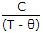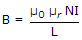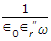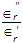# Online Electronics and Communication Engineering Test - Materials and Components TestLoading Test...

Instruction:

• This is a FREE online test. DO NOT pay money to anyone to attend this test.
• Total number of questions : 20.
• Time alloted : 30 minutes.
• Each question carry 1 mark, no negative marks.
• DO NOT refresh the page.
• All the best :-).

1.

The conductivity of intrinsic semiconductor is given by

A.
 eni(μp - μn)B.
 eni(μp + μn)C.
 ni(μp + μn)D.
 ni(μpμn)Explanation:

For intrinsic semiconductor,

Conductivity = (Electron charge) x (Number of charge carriers) x (Sum of mobilities of holes and electrons).

2.

The law J = σE, where J is current density, σ is electrical conductivity and E is field strength is

A.
 Ohm's lawB.
 Gauss lawC.
 Ampere's lawD.
 Biot-Savart lawExplanation:

It is another form of Ohm's law.

3.

Material which lack permanent magnetic dipoles are known as

A.
 paramagneticB.
 diamagneticC.
 ferromagneticD.
 ferrimagneticExplanation:

Diamagnetic materials have no permanent magnetic diploes.

4.

Assertion (A): Ferroelectric materials have spontaneous polarization.

Reason (R): Above curie temperature, ∈ =for ferro-electric materials.

A.
 Both A and R are true and R is correct explanation of AB.
 Both A and R are true but R is not correct explanation of AC.
 A is true but R is falseD.
 A is false but R is trueExplanation:

Both A and R are correct. Spontaneous polarization vanishes above ferroelectric curie temperature.

5.

Assertion (A): In a perfect capacitor, the current density is given by ω ∈0E0'rcos(ωt + 90°), where ∈r' is real part of dielectric constant.

Reason (R): In a perfect capacitor, dielectric losses are zero.

A.
 Both A and R are true and R is correct explanation of AB.
 Both A and R are true but R is not correct explanation of AC.
 A is true but R is falseD.
 A is false but R is trueExplanation:

If dielectric losses are zero, the current in capacitor leads the applied voltage by 90°.

Also imaginary part of is ∈r is zero.

6.

Assertion (A): For a solenoid having N turns,.

Reason (R): For all magnetic materials, B increases linearly with I.

A.
 Both A and R are true and R is correct explanation of AB.
 Both A and R are true but R is not correct explanation of AC.
 A is true but R is falseD.
 A is false but R is trueExplanation:

Increase of B with I is not linear. Hence R is false.

7.

Assertion (A): In a parallel plate capacitor having area of plate 1 m2 and plate separation 1 m, the capacitance C and resistance R are given by C = ∈0'r and R =where ω is frequency, ∈r' and ∈r" are real and imaginary parts of dielectric constant.

Reason (R): The imaginary part of electric constant is responsible for dielectric losses.

A.
 Both A and R are true and R is correct explanation of AB.
 Both A and R are true but R is not correct explanation of AC.
 A is true but R is falseD.
 A is false but R is true8.

Inductors used at V.H.F. are made using

A.
 air coreB.
 cast iron coredC.
 sheet steel coredD.
 thin film techniqueExplanation:

Thin film technique ensure low hysteresis and eddy current losses.

9.

In body centred cubic structure, comers and centre of the cube are occupied by identical atoms.

A.
 TrueB.
 False10.

A dielectric is subjected to alternating field. The dielectric losses are potential to

A.
 real part of dielectric constantB.
 imaginary part of dielectric constantC.
 both real and imaginary parts of dielectric constantD.
 either real or imaginary part of dielectric constantExplanation:

Absorption of energy is proportional to imaginary part of complex dielectric constant.

11.

Assertion (A): Dielectric losses are proportional to tan δ.

Reason (R): tan δ =where ∈r' and ∈r" denote the real and imaginary parts of ∈r.

A.
 Both A and R are true and R is correct explanation of AB.
 Both A and R are true but R is not correct explanation of AC.
 A is true but R is falseD.
 A is false but R is true12.

The units for electric dipole moment are

A.
 coulombsB.
 colomb-metreC.
 coulomb/metreD.
 coulomb/m2Explanation:

It is product of charge and distance.

13.

Assertion (A): The equation D = ∈0rE is applicable only to isotropic materials.

Reason (R): In polycrystalline materials, the directional effects are absent.

A.
 Both A and R are true and R is correct explanation of AB.
 Both A and R are true but R is not correct explanation of AC.
 A is true but R is falseD.
 A is false but R is true14.

When an electron moves at velocity v in combined electric and magnetic fields E and H, the Lorentz force F is F = - e(E + v x B).

A.
 TrueB.
 False15.

Assertion (A): Holes are majority carriers in p type semiconductor.

Reason (R): In p type semiconductor, the electrons produced by thermal agitation recombine with holes.

A.
 Both A and R are true and R is correct explanation of AB.
 Both A and R are true but R is not correct explanation of AC.
 A is true but R is falseD.
 A is false but R is true16.

If WF is the Fermi level, then in a metal at T = 0

A.
 all energy levels above WF filled, all those below WF are emptyB.
 all energy levels below WF are filled, all those above WF are emptyC.
 some energy levels, both below and above WF, are filledD.
 all energy levels below and above WF are filled17.

Assertion (A): Dielectric constant is independent of temperature unless the temperature is extremely high.

Reason (R): For normal temperatures the number of atoms per unit volume is constant.

A.
 Both A and R are true and R is correct explanation of AB.
 Both A and R are true but R is not correct explanation of AC.
 A is true but R is falseD.
 A is false but R is trueExplanation:

For normal temperatures the dielectric constant depends on the number of atoms per unit volume.

18.

The temperature at which some materials become superconductors is called

A.
 Transition temperatureB.
 Curie temperatureC.
 Neel temperatureD.
 Onnes temperature19.

In an homogeneous dielectric subjected to an electric field E, the dipole moment per unit volume is ∈0(∈r - 1) E.

A.
 TrueB.
 False20.

The probability of recombination of electrons and holes in a semiconductor is proportional to

A.
 density of electronsB.
 density of holesC.
 density of electrons and holesD.
 none of the above